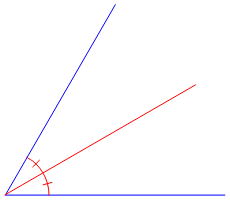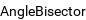# GET TUTORING NEAR ME!(800) 434-2582

/ Get Math Help

## Angle Bisector

IllustrationAlternate names
Definition

The (interior) bisector of an angle, also called the internal angle bisector, is the line or line segment that divides the angle into two equal parts. The angle bisectors meet at the incenter I, which has trilinear coordinates 1:1:1. The length t_1 of the bisector A_1 T_1 of angle A_1 in the above triangle Δ A_1 A_2 A_3 is given by t_1^2 = a_2 a_3[1 - a_1^2/(a_2 + a_3)^2], where t_i congruent (A_i T_i)^_ and a_i congruent (A_j A_k)^_. The points T_1, T_2, and T_3 have trilinear coordinates (0, 1, 1), (1, 0, 1), and (1, 1, 0), respectively, and form the vertices of the incentral triangle.

Related Wolfram Language symbol